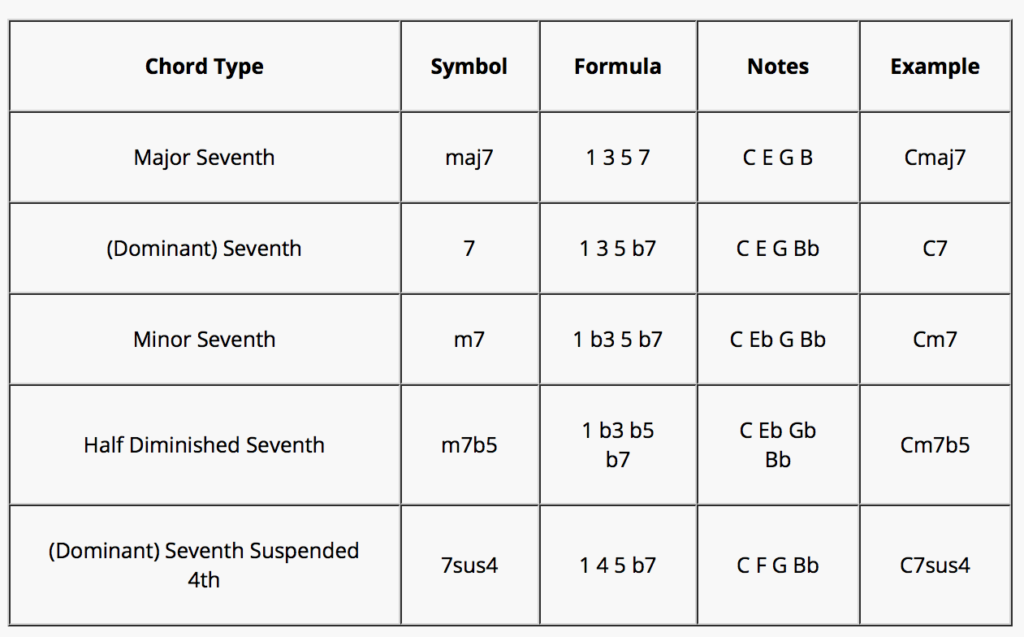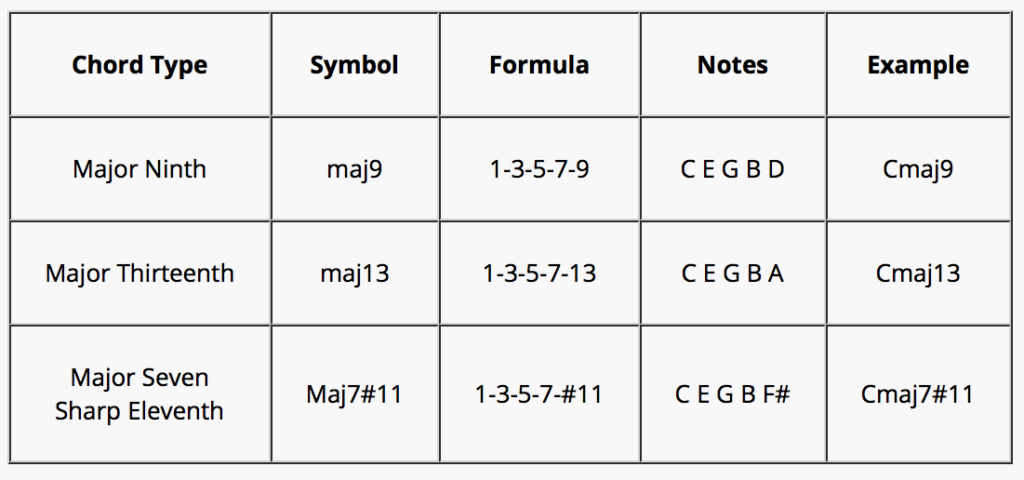Chord Formulas

Chord Formulas

Chord structures are represented by what we call “chord formulas”.
A chord formula is a combination of numbers, each number representing an intervallic relationship to the root of the chord, which is represented by the number 1.
The root of a chord, is the note that gives the chord it’s name. It’s the note the chord is “built” on.
The root of a C chord is the note C, the root of an Abm chord is the note Ab, the root of an F#m chord is the note F#, etc.

The root is always numbered as 1.
If the next note in the chord is up a 3rd from 1, then that will get the number 3.
If the next note is up a 5th from 1, then that note gets the number 5.

As an example, the notes that make up a C chord are C E G

C = 1
E = 3 (C D E … 1 2 3 –> E is up a 3rd from C)
G = 5 (C D E F G … 1 2 3 4 5 –> G is up a 5th from C)

This blog deals with chord formulas ll built from a root, not with inversions.
Things get a bit trickier with inversions.

For example, when you see following:

E G C

The formula for this is not: 1 b3 b6
This 1 b3 b6 formula would imply that E is the root (1) and that this hence is some kind of E chord.
Problem with that, is that this is not an E chord.
The notes C E G or any inversion of these 3 notes, always sounds like a C chord.

As such, the correct formula for the above would be 3 5 1 (E = the 3rd, G = the 5th and C = 1). This is a first inversion C chord, not an E chord.

How can you know?
It comes down to remembering (and then applying) a very important part in the study of harmony:

Chords are built stacking 3rd intervals!

Here’s how you apply this theory knowledge to figure out which chord the notes E G C add up to.

When I stack a 3rd up from “1st note” E, I get G (E F G). So far so good.
When I then stack another 3rd on top of G, I get B… not C. Conclusion: E G B are the notes that make up an E chord. Here we have E G C (a 3rd, E to G, and a 4th, G to C): This is not a C chord.

Maybe it could be a G chord.
Stacking a 3rd on top of G (because again: that is how you built chords), gives us the note B. Not a note in the above chord. Conclusion: this is not a G chord. (Stacking 3rd intervals on a G note gives us the notes G B D, which is what the notes are in a G chord)

There is only 1 possibility left: C

Stacking a 3rd on top of C gives us the note E (C D E… 1 2 3)
Stacking a 3rd up from E, gives us G. (E F G … 1 2 3)

C E G

Conclusion: the notes E G and C form a C chord. The notes are just out of order, which is what we call an inversion.
Put in ascending order starting from the root, we get C E G, and indeed: these notes are all 3rd intervals apart, which is how you built chords.

(On a side note: sus chords are built stacking 4th intervals.)

So as mentioned earlier: this blog doesn’t deal with inversions. That’s for a later blog.
The formulas of the inversions are much easier to understand and to figure out, once you have gotten all the upcoming chord formulas committed to memory.

This is the most thorough and indepth chord formulas list available on the internet.
Have fun!

Power Chord7th Chords (in the major scale)More 7th chords6th Chords6th chords are always chords with a major 6th interval, never a minor 6th interval.
When you add a b6 to a 1 3 5 or 1 b3 5 triad, that b6 takes over and feels like the root.
This becomes clear when you add note names to the numbers of the formula, as shown in following examples:

1. 1 3 5 b6 = C E G Ab = 1st inversion of Ab C E G
This sounds like a 1st inversion Abmaj7#5 chord more than it sounds like a C chord

2. 1 b3 5 b6 = C Eb G Ab = 1st inversion of Ab C Eb G
This sounds like a 1st inversion Abmaj7 chord, more than it sounds like a C chord.Add 11 is quite a clashy, nasty sounding chord, with the E and the F note adding a lot of tension.
I added it here for thoroughness, but I don’t think I’ve ever encountered this chord in any song.

maj7maj7#11 happens on the IV chord in a major scale. This chord has a Lydian sound.

maj7 with Tension CombinationsIn chords with lots of tensions, you usually leave lesser important notes out, like the 5th or even the root.

m7m7 Tension CombinationsDominant 7Dominant chords (1 3 5 b7) with what you call altered tensions b5 #5 b9 #9, are called “altered chords”
The altered chord is chord I in the altered scale. The altered scale is the 7th mode of melodic minor.
What this means is that when you play a melodic minor scale starting on note number 7 of that scale, you are then playing an altered scale. The first chord in the altered scale is the altered chord.

m7b5The “6/9” chordsConclusion

Hit me up anytime at [email protected] if you have any questions, or if you would like to book a lesson.

Keep me informed on your progress. You can hit me up in the comments section below.
If you like this blog: give it a rating and feel free to also give me any feedback.
I believe everything can always be improved. I’d gladly implement your suggestions and ideas in this blog or the next.

Be on the look out for more blogs about everything guitar, music, songwriting and music education.
You’re on your way to becoming a great guitar player.
Have fun! 🙂(1 votes, average: 5.00 out of 5)